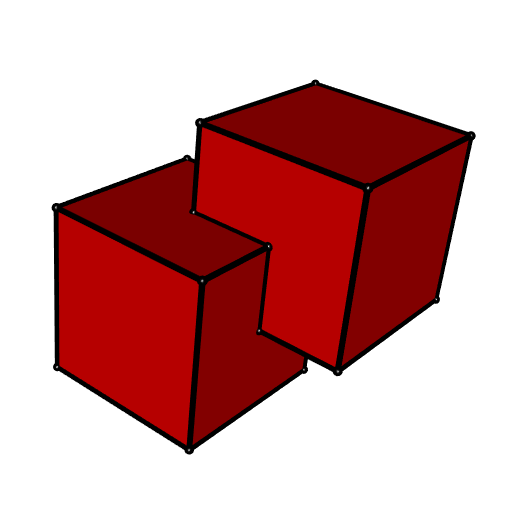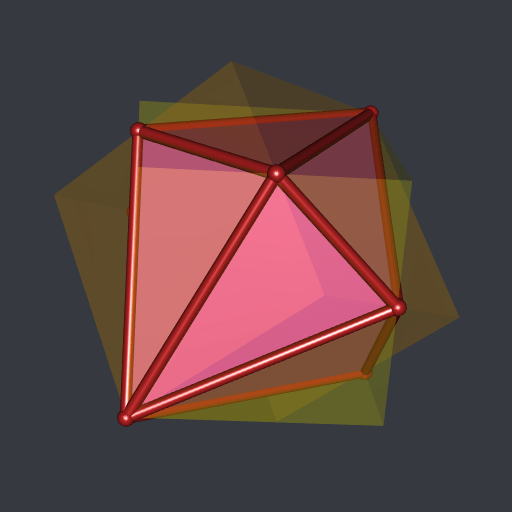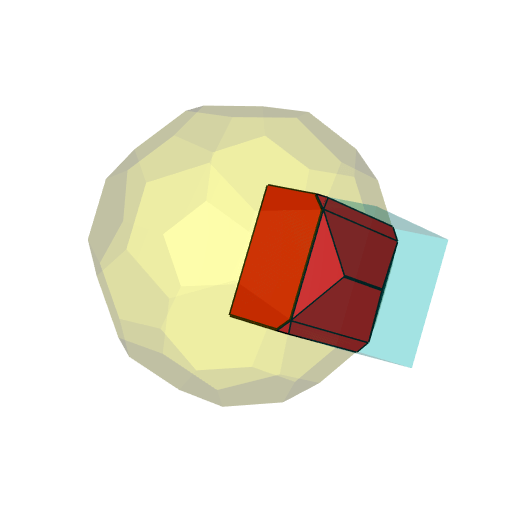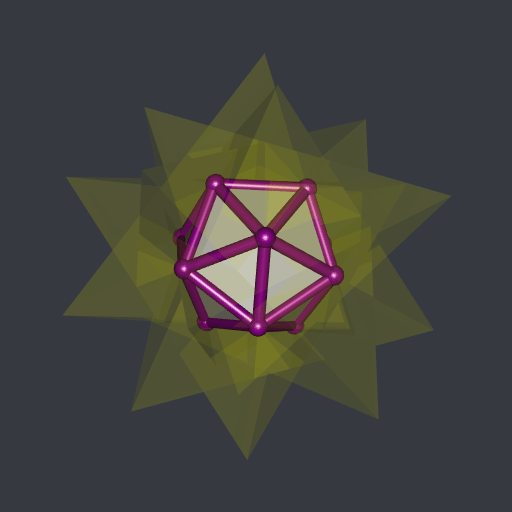Want to share your content on R-bloggers? click here if you have a blog, or here if you don't.

I?m still working on my package RCGAL, that I already present in a previous post.

This previous post was about the reconstruction of surfaces. Now I implemented the Boolean operations on meshes. Here are some simple examples.

#### Union of two cubes:#### Intersection of two cubes (one rotated):#### Difference of two cubes:#### Intersection of a cube and a truncated icosahedron:The code generating these plots is given in the RCGAL examples.

Now let?s turn to a more interesting example.

## The compound of five tetrahedra

The compound of five tetrahedra is provided by RCGAL. These are five tetrahedra in a pretty configuration, each centered at the origin. You can get their meshes by typing `tetrahedraCompound`. This is a list with two components: a field `meshes` providing for each tetrahderon its vertices and its faces, and a field `rglmeshes`, similar to `meshes` but these meshes are ready for plotting with the rgl package. Here it is:

```library(RCGAL)
library(rgl)
rglmeshes <- tetrahedraCompound[["rglmeshes"]]
open3d(windowRect = c(50, 50, 562, 562), zoom = 0.75)
bg3d("#363940")
colors <- hcl.colors(5, palette = "Spectral")
invisible(lapply(
1:5, function(i) shade3d(rglmeshes[[i]], color = colors[i])
))```I wondered for a long time what is the intersection of these five tetrahedra. But I didn?t have any tool to compute it. Now I have. Let?s see.

```# compute the intersection ####
inter <- MeshesIntersection(
tetrahedraCompound[["meshes"]], numbersType = "lazyExact", clean = TRUE
)
# plot ####
open3d(windowRect = c(50, 50, 562, 562), zoom = 0.75)
bg3d("#363940")
# first the five tetrahedra with transparency ####
invisible(lapply(
rglmeshes, shade3d, color = "yellow", alpha = 0.1
))
# now the intersection ####
rglinter <- tmesh3d(
"vertices"    = t(inter[["vertices"]]),
"indices"     = t(inter[["faces"]]),
"homogeneous" = FALSE
)
# and finally the edges ####
plotEdges(
inter[["vertices"]], inter[["exteriorEdges"]],
only = inter[["exteriorVertices"]], color = "darkmagenta"
)```

Here is the result:This is an icosahedron, I think.

Unfortunately, R CMD CHECK still throws some warnings which prevent me to publish this package on CRAN. I hope this issue will be solved.